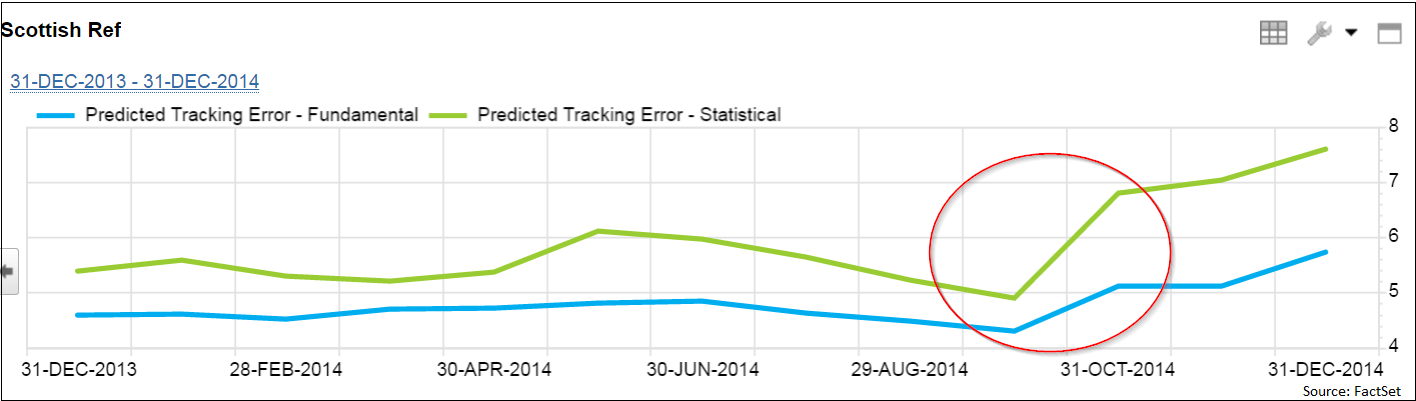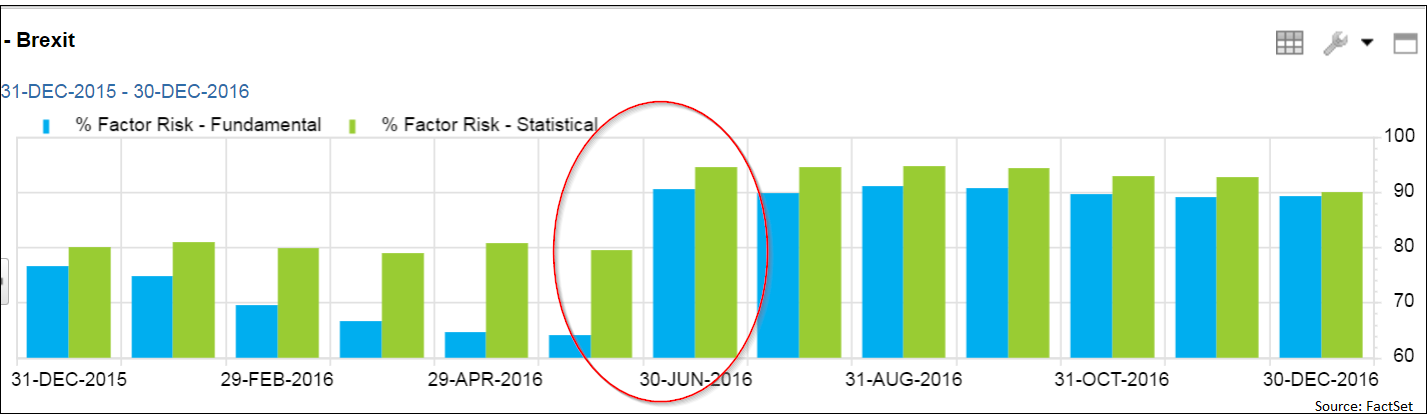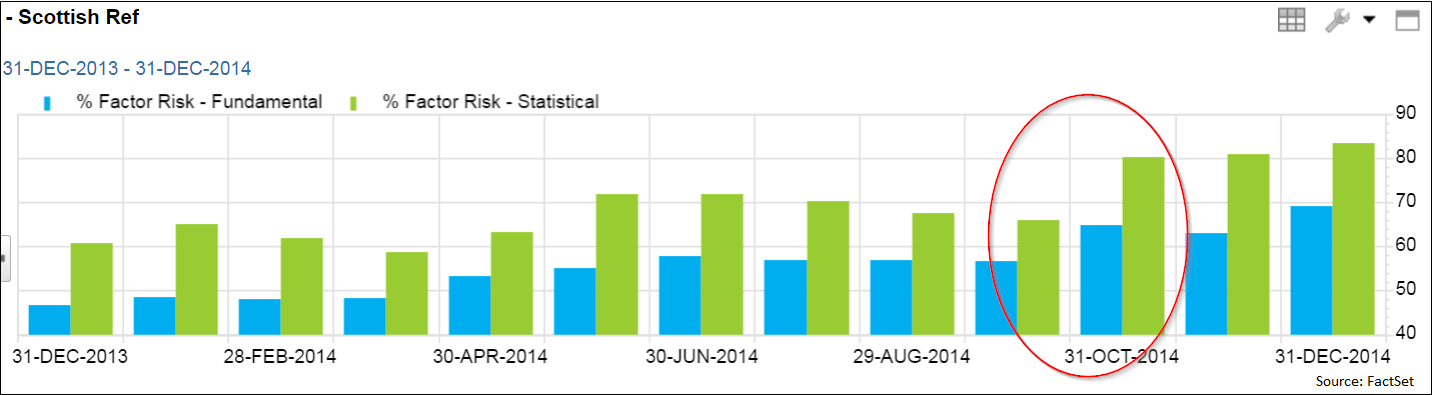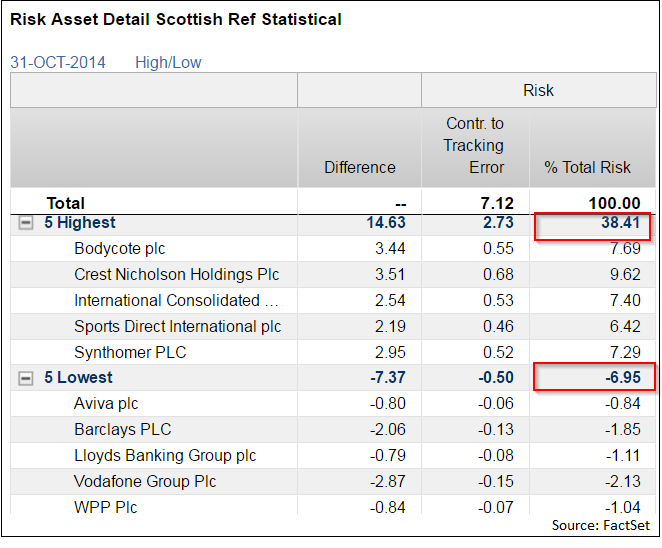# The Fundamental Differences Of Fundamental And Statistical Risk Models

Fundamental risk models are commonly used by investment professionals to manage risk for their portfolios, primarily due to the ease of interpretation on an ongoing basis. However, statistical risk models provide an interesting alternative insight into the risk dynamics of a portfolio, especially during unpredictable market movements.

## Get Our Activist Investing Case Study!

Get the entire 10-part series on our in-depth study on activist investing in PDF. Save it to your desktop, read it on your tablet, or print it out to read anywhere! Sign up below!

Statistical risk models, unlike fundamental risk models, are not restricted to the fixed set of pre-defined factors. Rather, statistical models use a more dynamic approach to decompose the risk into a given set of self-adapting factors. In effect, such sharp adaptability means that statistical factors model risk in a way that explains those dimensions of risk that the fundamental factor model captures as the idiosyncratic component of risk. However, these dynamic statistical model factors lack the intuitive interpretation of fundamental model factors, making risk decomposition and attribution analysis difficult to practically implement.

# Notes From Schwarzman, Sternlicht, Robert Smith, Mary Callahan Erdoes, Joseph Tsai And Much More From The 2020 Delivering Alpha ConferenceThe following are rough notes of Stephen Schwarzman, Steve Mnuchin, and Barry Sternlicht's interview from our coverage of the 2020 CNBC Institutional Investor Delivering Alpha Conference. We are posting much more over the next few hours stay tuned. Q2 2020 hedge fund letters, conferences and more One of the most influential investor conferences every year, Read More

In this article, I have taken two prominent British events, the Brexit vote and the Scottish referendum, to highlight the application of a statistical risk model in parallel with a fundamental factor model to gain additional cognizance of the risk outline for a fund and improve upon the investment process as a whole.

### Overview of Statistical Risk Models

A statistical risk model has its factors constructed using principal component analysis (PCA), which ensures that those factors have the maximum explanatory power by processing asset return time series. This technique dynamically selects factors based on the maximum commonality among asset returns rather than constraining the model on a set of pre-defined factors.

To elaborate further, the use of the PCA technique enables the formulation of statistical model factors (principal explanatory component, in this case) by clustering securities in sets in order to maximize asset return correlation within the cluster. At the same time, the clustered securities will have negligible correlations with the rest of the securities’ returns, thus enabling the derived factors to capture maximum risk.

Mathematically, both fundamental and statistical risk models begin with the same linear factor model of asset returns:

R = Bf + u  ................................................... (1)

R is a vector of asset returns, B is a matrix of factor exposures, f is a vector of factor returns, and u is a vector of asset-specific, idiosyncratic returns. While R is known, fundamental and statistical risk models approach the solution of the rest of the terms in this equation differently.

While fundamental models have the factors and their exposures (B) given, the equation is solved for the factor return, f, using regression; in macroeconomic models, the returns are available while the factor exposures are estimated. However, for statistical risk models, both the matrix of factor exposures, B, and the vector of factor returns, f, are solved for simultaneously to maximize the predictive power of the above equation.

In a nutshell, the factors of the statistical model showcase high adaptability of the factors, especially relevant in times when the market is predominantly driven by unexpected factors or extreme events.

### Case Study

The vote for Scottish Independence and Brexit present classic instances for assessing high market uncertainty, which was ubiquitous at the time. A stark divide amongst the electorate on both issues made it extremely difficult for political pundits to predict the outcome of these votes. This high level of unpredictability added to the uncertainty of the performance of financial markets and unsettled economic conditions in the British Isles.

To understand the outcome of these events, we consider a fund with exposure to the UK universe of companies and benchmarked to the FTSE All Cap UK, comparing the results from Axioma’s monthly statistical and fundamental models on FactSet.

### Leveraging Statistical Risk Models - Active Risk Profiles at fund level

Considering the charts (Figure 1 and Figure 2) below, we observe a significant rise in the tracking error coinciding with the periods when the events took place, indicating the presence of high volatility in the market.

Figure 1

Figure 2It also appears that a relatively higher proportion of the prevailing volatility is captured by the statistical model (green line). The derived spread between the tracking error captured by the statistical model and the fundamental could give further insights on the risk profile of the portfolio in such scenarios.

For reference, Stat Minus Fund Risk Spread here equals the predicted risk from the statistical model minus the predicted risk from the fundamental model with the same estimation universe.

### Risk Models Decomposition - Factor vs.Specific Risk

Risk Analysis, in a traditional setup, is broken down into two major components: the risk explained by the model factors (Factor Risk), and the part which is not explained by the factors captured as the Stock Specific (idiosyncratic) risk.

The factors of a statistical model are derived by isolating a set of securities from the estimation universe having the maximum asset return correlation amongst themselves whilst having negligible correlations with the rest of the securities returns. Factors thus derived from various similar clusters of securities are able to capture greater volatility than the factors of a conventional fundamental model.

Using FactSet’s multi-tile charting in PA3.0, a simultaneous analysis (Figure 3 and Figure 4) clearly illustrates that although both the model variants showcase a similar trend for the “% Factor Risk” for their respective time horizons, the statistical model seems to be picking up a factor missing from the fundamental model’s factor risk profile. This can be captured as a spread between the two “% Factor Risk” metrics at the top level.

Figure 3In the case of Brexit, there was substantial increase in market uncertainty during the buildup to voting day. This is primarily attributed to closely fought campaigns and polarization stemming from pollsters’ inability to predict the outcome of the referendum confidently. This factor risk is captured by the high “% Factor Risk” spread between the two model variants. However, following the declaration of the results, the magnitude of the market uncertainty gradually came down, resulting in a lower “% Factor Risk” spread.

Figure 4In contrast, when we look at the Scottish referendum, we can see that the pre-event risk spread is relatively narrow compared to the wider post-event risk spread. The closely fought decision resulted in greater devolution of powers from the UK government to the Scottish Government as well as build-up of regional sentiment towards a second referendum for independence. These post-event factors in the aftermath of the vote led to increased market uncertainty (although for a short period), resulting in the widening of the factor risk spread post event day.

### Analyzing Risk Granularity - Asset Level Decomposition

Looking at these scenarios, the ability to decompose the total risk at an asset level to assess the drivers of risk in terms of the asset’s contribution to risk is obviously beneficial to investors. The term “% of total Risk” would decompose the total tracking error in terms of its contribution from each asset based on the asset’s weight and riskiness.

In a typical setup, a stock picker would tend to accumulate more of the specific risk component rather than factor risk by placing bets based on picking individual stocks. On the contrary, a quintessential market timer would tend to pick more factor risk rather than the specific risk, as the risk model factors would represent the market trends at the given time.

In the case of the Scottish Referendum, let’s consider the top and bottom five contributors to risk in the fund as per the statistical and fundamental models.  Where the top five names account for more than 27% of the risk budget as per the fundamental model, they account for almost 40% as per the statistical model.

Figure 5Similarly, the bottom five names diversify away close to 7% of the total risk in case of the statistical models.

In both instances, we can see that these names are inherently risky (in the sense of either diversification or concentration). Moreover, the prediction of how risky they are is uncertain due to the varying amounts of risk contribution coming from the two model variants.

Figure 6Hence, a portfolio manager may consider re-aligning the exposures to these positions if he does not have enough conviction in the securities. This is similar to managing factor exposures—they should not be large (or small) unless the portfolio manager wants them to be large (or small) as a part of the fund’s mandate or strategy.

Fundamental models are suitable in situations already incorporating management of factor exposures and risk-based attribution, while statistical models have a relative edge in picking up short-term market uncertainty and translating it into risk. It is important to understand the applicability of each model’s variants given the nature of the analysis, and how this would in effect help better utilize the models - either independently or together in parallel to widen the scope of the analysis.

Article by By Mrudul Neralla, FactSet